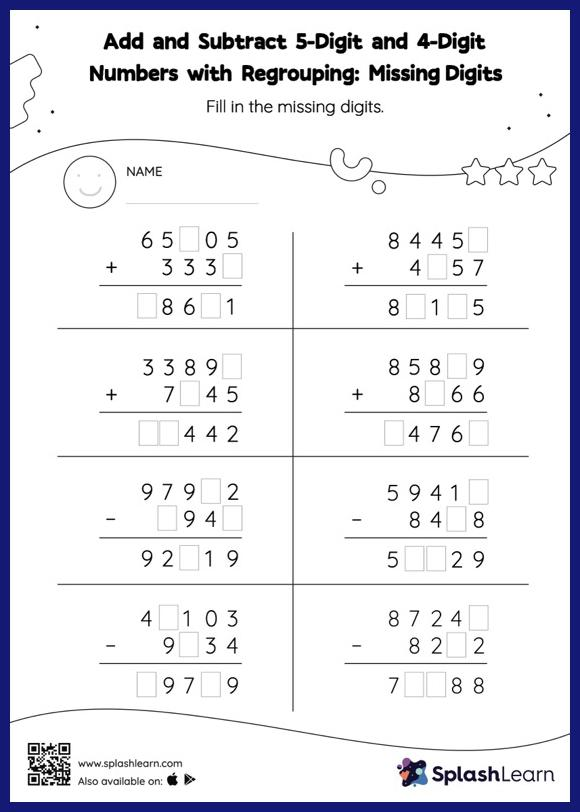# Add and Subtract 5-Digit and 4-Digit Numbers with Regrouping: Missing Digits Worksheet

Home > Add and Subtract 5-Digit and 4-Digit Numbers with Regrouping: Missing DigitsChildren practice adding and subtracting 5-digit and 4-digit numbers with regrouping with this worksheet. In this worksheet, students must find the missing number using the relationship between addition and subtraction. To solve add and subtract 5-digit and 4-digit numbers with regrouping worksheet, they must also regroup the numbers. This worksheet is about practicing the column method in which numbers are written one on top of another. In this method, students use their place value understanding to solve the problems.